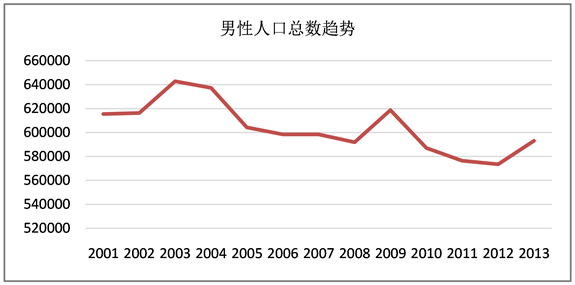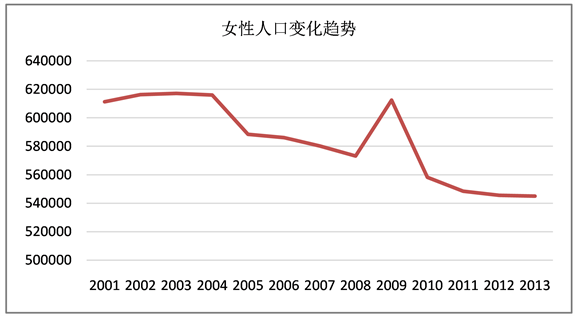﻿ 延迟生育对人口的影响

延迟生育对人口的影响The Effect of Delayed Birth on the Population

Abstract: Aiming at the problem of the influence of the delayed birth on the population, this paper uses the enumeration method, the Weber distribution theory and the grey forecast method to construct the population forecast model based on Leslie, the birth rate model based on Weber distribution and the grey forecast model, etc. It is concluded that the birth age of the first child will be advanced after the adjustment of the birth policy, the change of the birth policy will skew the sex ratio of the youth in the future, and the number of births per year will not increase too much after the opening of the three-child policy.

1. 引言

2. 模型分析与计算

2.1. 问题一的分析与求解

2.1.1. 对问题一的分析

2.1.2. 对问题一的求解Table 1. Comparison of fertility rates among women of all ages in 2006~2015

2.2. 问题二的分析与求解

2.2.1. 对问题二的分析

2.2.2. 对问题二的求解

1) 模型准备

2) 模型的建立

$X\left(t+1\right)=LX\left(t\right)$

L是指Leslie矩阵，n为将人口按年龄划分的n个级别。

${p}_{i}=\frac{{x}_{i+1}\left(t+1\right)}{{x}_{i}\left(t\right)}，\left(i=1,2,\cdots ,n\right)$

${x}_{0}\left(t+1\right)=\underset{i=0}{\overset{n}{\sum }}{b}_{i}\left(t\right){w}_{i}\left(t\right){x}_{i}\left(t\right)$

$\begin{array}{l}{x}_{0}\left(t+1\right)=\underset{i=0}{\overset{n}{\sum }}{b}_{i}\left(t\right){w}_{i}\left(t\right)\\ {x}_{i}\left(t+1\right)={x}_{i}\left(t\right){p}_{i}\end{array}$

$j>n-1$ 时， ${p}_{j}$ 均为0，即某种种群达到第n年龄级后便会全部死亡，则：

${p}_{j}=1-\frac{第七年内第\text{ }i\text{ }年龄级的死亡人数}{第七年内第\text{ }i\text{ }年龄的人口数}$

3) 模型的求解Figure 1. Trends in the overall male populationFigure 2. Trends in the overall female population

1) 模型的建立

2) 模型的求解

$g\left(x\right)=a×b{\left(x-{x}_{0}\right)}^{b-1}×{\text{e}}^{\left[-a{\left(x-{x}_{0}\right)}^{b}\right]}$

1) 模型的建立

${x}^{0}\left(1\right),{x}^{0}\left(2\right),\cdots ,{x}^{0}\left(M\right)$ 是所要预测的某项指标的原始数据。对原始数据作一次累加生成处理，即

${x}^{\left(1\right)}\left(M\right)=\underset{i=1}{\overset{M}{\sum }}{x}^{\left(0\right)}\left(t\right)$

$\frac{\text{d}{x}^{\left(1\right)}}{\text{d}t}+a{x}^{\left(1\right)}=u$

$\left[\begin{array}{c}a\\ u\end{array}\right]={\left({B}^{\text{T}}B\right)}^{-1}{B}^{\text{T}}{Y}_{M}$ .

$B=\left[\begin{array}{cc}-\frac{1}{2}\left[{x}^{\left(1\right)}\left(1\right)+{x}^{\left(1\right)}\left(2\right)\right]& 1\\ -\frac{1}{2}\left[{x}^{\left(1\right)}\left(2\right)+{x}^{\left(1\right)}\left(3\right)\right]& 1\\ ⋮& ⋮\\ -\frac{1}{2}\left[{x}^{\left(1\right)}\left(M-1\right)+{x}^{\left(1\right)}\left(M\right)\right]& 1\end{array}\right]$

2) 求出预测模型

${x}^{\left(1\right)}\left(t+1\right)=\left[{x}^{\left(0\right)}\left(1\right)-\frac{u}{a}\right]{\text{e}}^{-at}+\frac{u}{a}$

3) 模型的求解

${x}^{\left(0\right)}\left(t\right)=\left[138271,137462,136072,135404,134735,134091\right]$

${x}^{\left(1\right)}\left(M\right)=\underset{i=1}{\overset{M}{\sum }}{x}^{\left(0\right)}\left(t\right)$ 式得一次累加数据序列

${x}^{\left(1\right)}\left(t\right)=\left[138271,275733,411805,547209,681944,816035\right]$

$\begin{array}{l}{B}^{\text{T}}=\left[\begin{array}{ccccc}-207002& -156767& -4799501& -6145765& -7489895\\ 1& 1& 1& 1& 1\end{array}\right]\\ {Y}_{M}={\left[\begin{array}{ccccc}275733& 411805& 547209& 681944& 816035\end{array}\right]}^{\text{T}}\end{array}$

$\left[\begin{array}{c}a\\ u\end{array}\right]=\left[\begin{array}{c}-0.00073\\ 2.0957\end{array}\right]$

${x}^{\left(1\right)}\left(t+1\right)=2965.04{\text{e}}^{-0.00073t}-2870.82$

2.3. 问题三的分析与求解

2.3.1. 对问题的分析

2.3.2. 对问题的求解

$t{\int }_{a}^{b}\left(j,t\right)\text{d}t={\int }_{a}^{b}\frac{\left(j,t\right)}{{p}_{a}^{b}\left(j,t\right)}\text{d}t,\text{\hspace{0.17em}}\text{\hspace{0.17em}}\eta \le j\le \psi$

3. 结论

1) 仅开放三胎政策效果并不明显，因为即使政策对城市地区有效，但对乡镇效果并不明显，总体而言，开放三胎政策后每年新生儿增加也不会太多。

2) 新生儿男女性别比例失衡现象是近年来才出现的，而且非常严重，所以越宽松的生育政策，使得青年性别比例在未来越失衡。

3) 根据问题一的结论得知，即使开放三胎政策之后对新生人口的影响并不大，但是还是会有许多家庭考虑到以后会生育更多子女，所以第一胎的生育年龄还是会提前的。

 王广州. 生育政策调整研究中存在的问题与反思[J]. 中国人口科学, 2015(2): 2-15.

 宋全成, 文庆英. 我国单独二胎人口政策实施的意义、现状与问题[J]. 南通大学学报(社会科学版), 2015, 31(1): 122-129.

 叶文振. “单独二胎”生育政策的女性学思考[J]. 中共福建省委党校学报报, 2014(12): 58-63.

 李涌平, 蔡天骥. 理解单独二胎人口政策: 为了未来人口发展的平衡[J]. 人口与发展, 2014, 20(6): 104-109.

 邓爽, 刘杰, 贺雪娇. 我国实施“全面二胎”生育政策的利弊分析[J]. 产业与科技论坛, 2016, 15(4): 7-8.

 王金营, 戈艳霞. 全面二孩政策实施下的中国人口发展态势[J]. 人口研究, 2016, 40(6): 3-21.

 张丽萍, 王广州. “单独二孩”政策目标人群及相关问题分析[J]. 社会学研究, 2014, 29(1): 25-39.

 王军. 生育政策调整对中国出生人口规模的影响——基于生育意愿与生育行为差异的视角[J]. 人口学刊, 2015, 37(2): 26-33.

Top